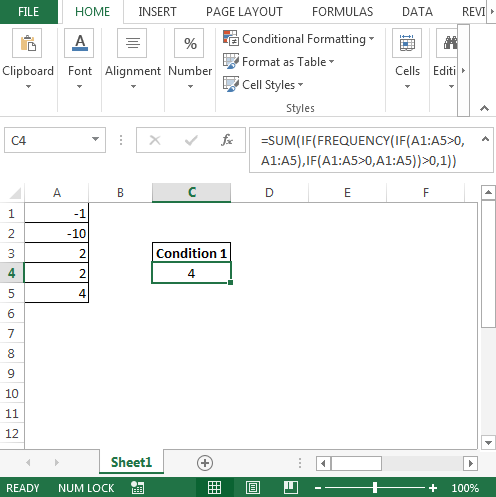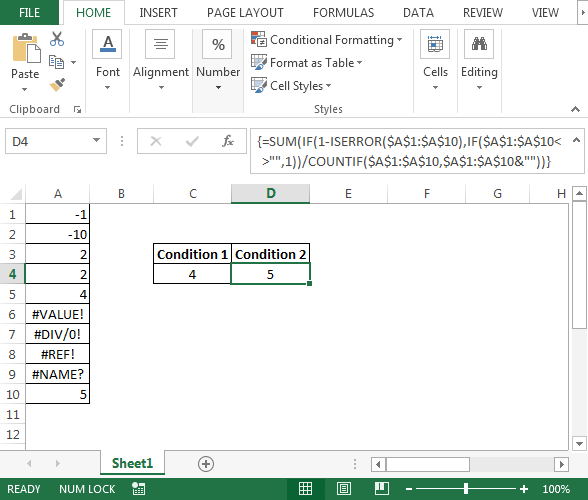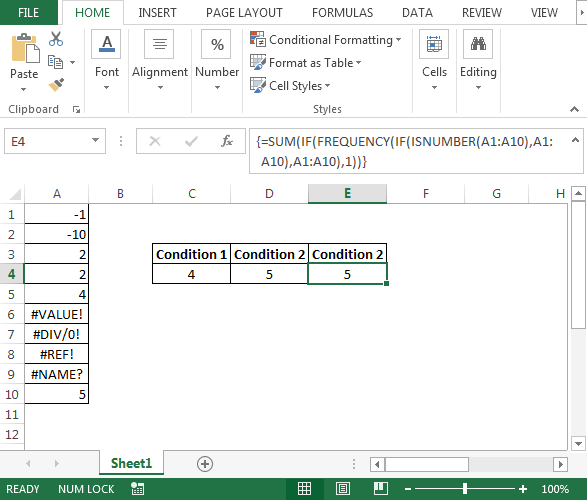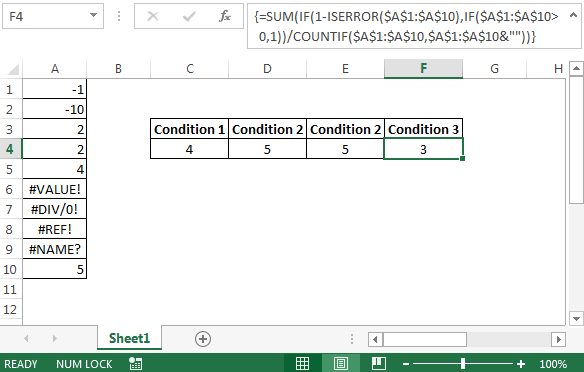# How to extract unique numbers from a range in Microsoft Excel 2010

In this article, we will discuss how we can identify unique numbers in Microsoft Excel using formulas.

There can be various scenarios. However, we will cover the common problems that users usually face in their daily work.

The problems can be of following types:

1. There can be negative numbers or positive numbers in the range.

Answer should be count of unique values in Excel.

1. There can be negative numbers, positive numbers & errors like #REF!, #VALUE!, #DIV/0!, #NAME?in the range.

Answer should be count of unique identification number by ignoring / skipping any error type.

1. There can be negative numbers, positive numbers & errors like #REF!, #VALUE!, #DIV/0!, #NAME? in the range.

Answer should be count of unique positive numbers, ignoring any error type.

Example 1

Count of unique numbers from negative & positive numbers.

Following is the snapshot of data we have wherein number 2 is repeated twice & we want formula to count number 2 as one:

• The formula in cell C4 is
• =SUM(IF(FREQUENCY(IF(A1:A5>0,A1:A5),IF(A1:A5>0,A1:A5))>0,1))Example 2

Count of unique numbers from negative, positive numbers & errors i.e. #REF!, #VALUE!, #DIV/0!, #NAME? in the range.

• The formula in cell D4 is
• {=SUM(IF(1-ISERROR(\$A\$1:\$A\$10),IF(\$A\$1:\$A\$10<>””,1))/COUNTIF(\$A\$1:\$A\$10,\$A\$1:\$A\$10&””))}

Note: This is an array formula. CTRL + SHIFT + ENTER keys need to be pressed together.The same result can be achieved by applying a combination of SUM, IF, ISNUMBER, FREQUENCY.

• The formula in cell E4 is
• {=SUM(IF(FREQUENCY(IF(ISNUMBER(A1:A10),A1:A10),A1:A10),1))}

Note: This is an array formula. Use CTRL+ SHIFT + ENTER keys together to get result.Example3

Count of unique positive numbers after ignoring any error type in the range.

• The formula in cell F4 is
• {=SUM(IF(1-ISERROR(\$A\$1:\$A\$10),IF(\$A\$1:\$A\$10>0,1))/COUNTIF(\$A\$1:\$A\$10,\$A\$1:\$A\$10&””))}

Note: This is an array formula. Use CTRL+ SHIFT + ENTER keys together to get result.In this way, we can get the Excel count of unique numbers after meeting various conditions.Terms and Conditions of use

The applications/code on this site are distributed as is and without warranties or liability. In no event shall the owner of the copyrights, or the authors of the applications/code be liable for any loss of profit, any problems or any damage resulting from the use or evaluation of the applications/code.